Group assignment¶

There are different architectures of microcontrollers that we can find on the market.

However, for this week’s assignment, we can compare two different architectures, CISC and RISC.

CISC is Complex Instruction Set Computing which relates to X86 processors. The purpose of this architecture is to resolve multiple complex operations at the same moment.

RISC - Reduced Instruction Set Computing relates to more simple logic and does not support resolving complex tasks. This architecture usually associated with ARM microcontrollers produced by Atmel company.

Despite the fact, these microcontrollers cannot be used for very complex tasks. These microcontrollers have certain advantages:

• the prices are much lower
• the power consumption is lower
• these microcontrollers are more efficient for the systems that require providing one operation at the moments
• these microcontrollers can be easily implemented for simple functions

The individual assignment¶

The goal was to make the code that will allow the user to increase the blinking speed with the external dimmer. For this task, we should use function MAP that will convert the analog signal into the pattern that we need.

Some things that I have learned from the data sheet. The most important and useful for me is that the Analog pins are dedicated to receiving the analog signal from the input devices such as a potentiometer.

The way it works is simple; the microcontroller read that signal on the analog pin. The signal is the fluctuation of the voltage. For example, the potentiometer gives from 0 to 5 volts. The AtTiny receives this signal and evaluates it in the way determined by the program. For example, the program may say, convert the voltage into a certain number from 0 to 255. The 0 volts will mean 0, and 5 volts will mean 255.

However, it is interesting how AtMega evaluates the analog signal. For example, in case AtMaga works with 5 volts, and it receives 4 volts. Then it understands the difference between its voltage and received voltage and gives a certain result.

Nevertheless, the voltage in the circuit is not stable. For example, at some moments, it can become lower. In case AtMega still receives 4 volts on the analog pin, the difference between AtMega voltage and receiving voltage becomes smaller. The measure can be effected in such situations.

Therefore, in case we use AtMega and receive an analog signal, it is better to have a stable voltage benchmark. The developers of AtMega made a special AREF pin for this purpose. It is necessary o connect the small capacitor to this pin with the GND on the other side. In this case, if the voltage fluctuates, the capacitor can give a certain voltage to the AREF pin, so the AtMega always understand the right level of voltage and can compare it with receiving signal.

First, we need to define the pins that we will use for this task.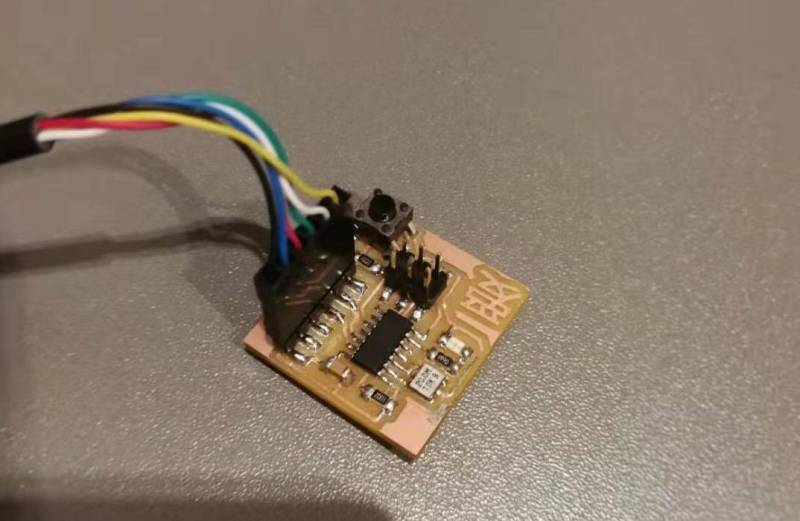As we can see, there are free pins, except those that we use to connect FabISP. Luckily we also can use those pins in case we do not connect the programmer. I decided to use the pin PA5 (Analog, Input) to receive the signal from the dimmer. The whole scheme should look in this way. Other two pins of dimmer should be connected to “GND” and “VCC.”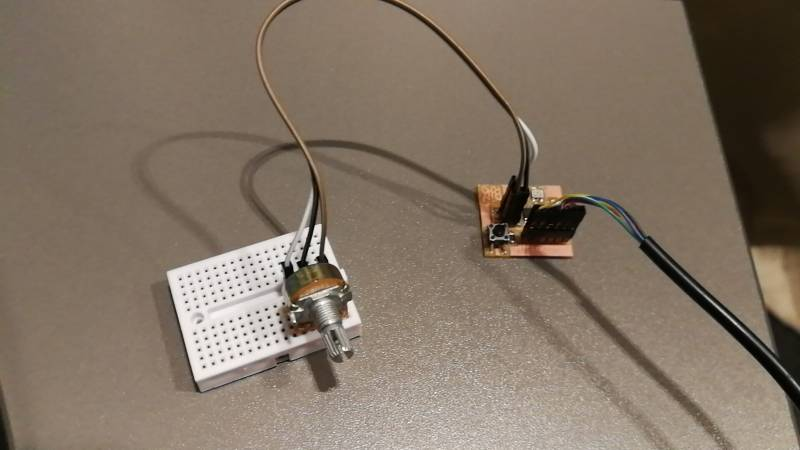However, before actual programming, we should connect the board to FabISP.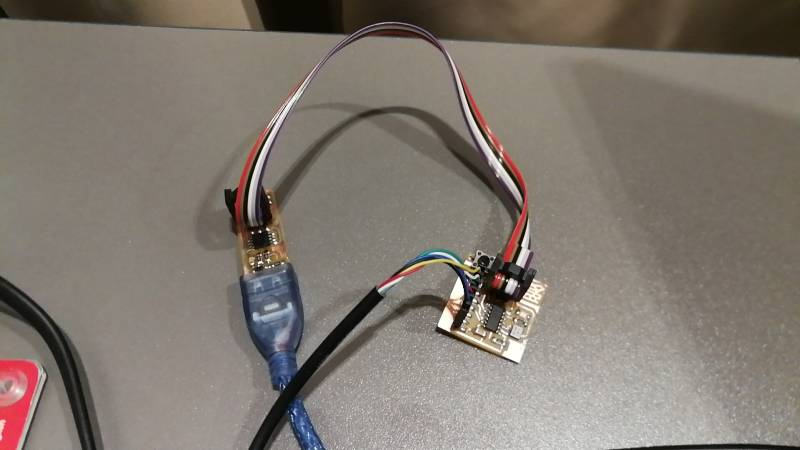I have used the Arduino terminal to program the board. In this case, we should set up all necessary features of the programming board.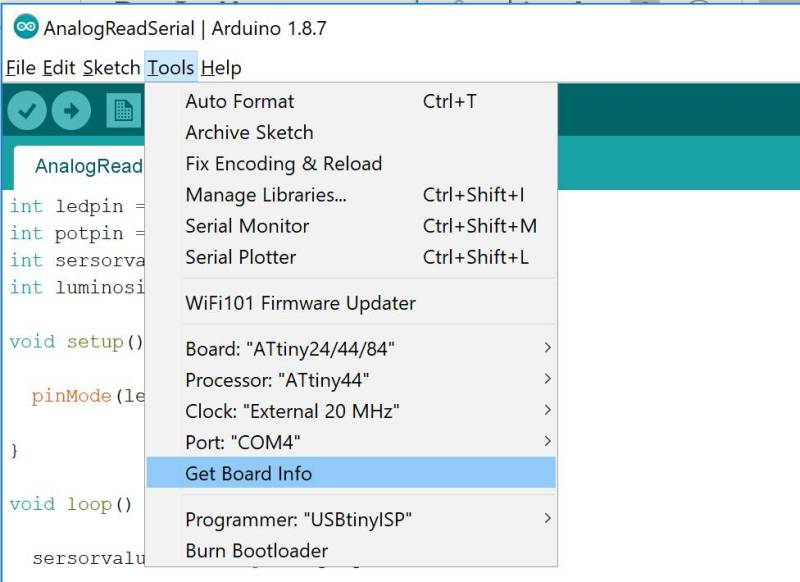As the pin numbers on ATtiny and Arduino board are different, it is necessary to understand what numbers to use when writing the code. I have used the following scheme to locate the necessary pins.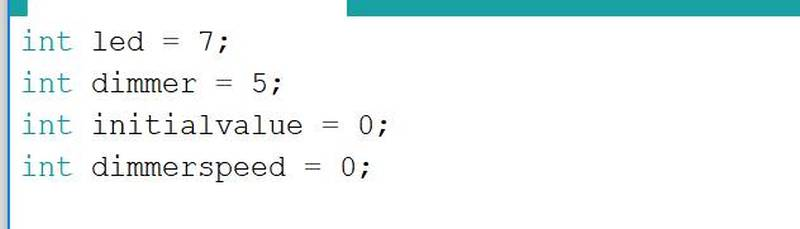For example, as we can see the pin number 6 on ATTiny44/84 is pin number 7 on Arduino. It means that while we create the code in Arduino terminal, we should use number 7 to link pin number 6 on ATTiny.

Next, we need to define pin on ATTiny that will be used in our code.

In my case it was:

• Pin number 6 (Arduino is 7) on ATTiny is LED.
• Pin number 10 (Arduino is 3) on ATTiny is a button.
• PA5 pin in number 8 on ATTiny and 5 on Arduino.

On the next step, we can start writing the code. First, we need to define the primary datatype for number storage.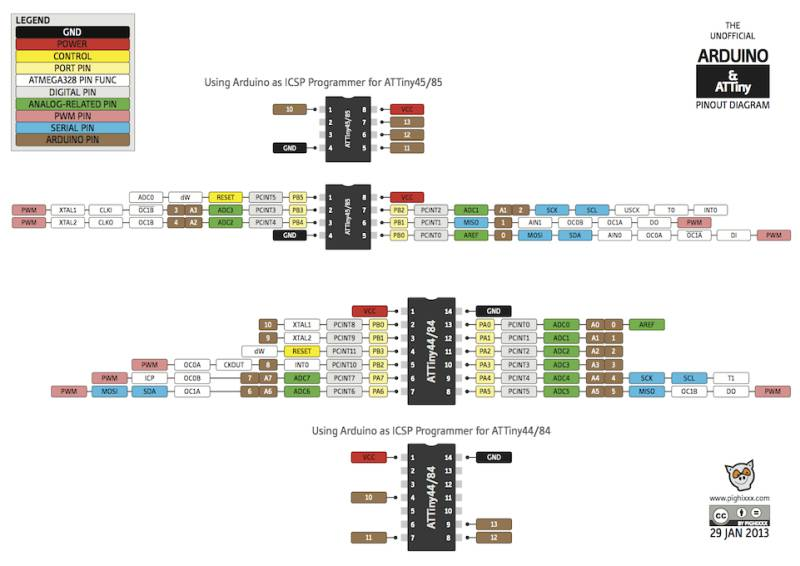Then we define the type of pins INPUT or OUTPUT.And finally, we write the actual process.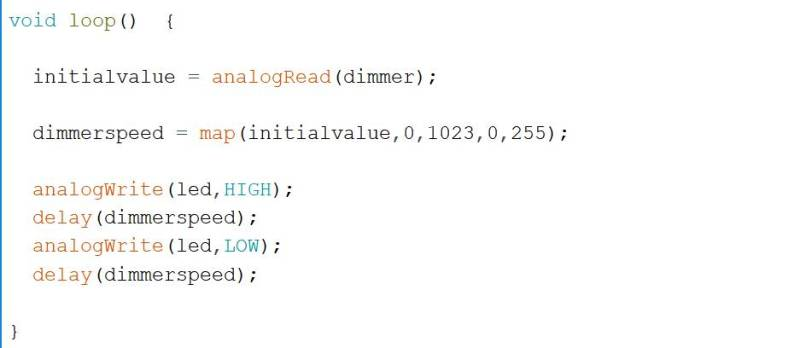The whole code is here:

int led = 7;
int dimmer = 5;
int initialvalue = 0;
int dimmerspeed = 0;

void setup() {

pinMode(led,OUTPUT);
pinMode(dimmer,INPUT);
}

void loop()  {